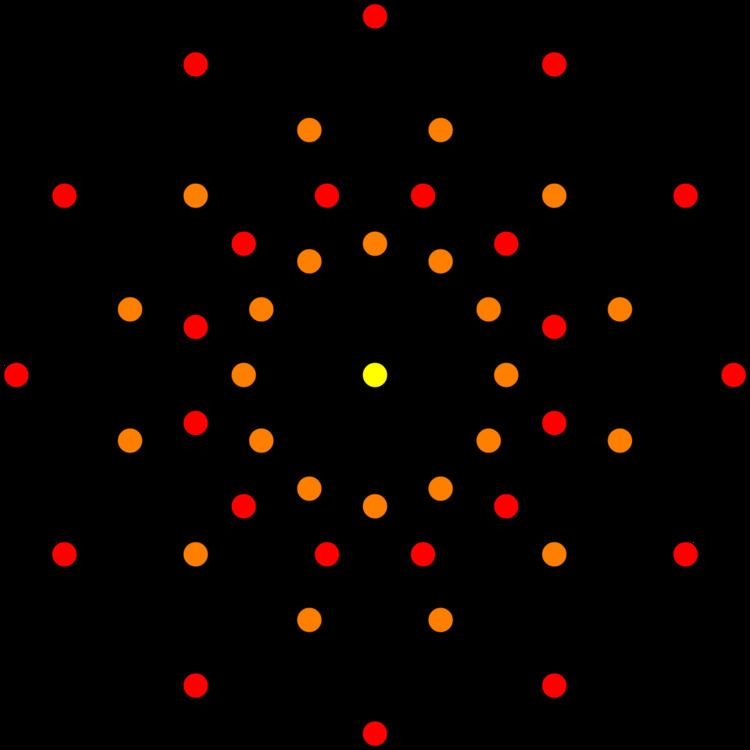# 1 42 polytope

Updated on
Edit
Like
CommentIn 8-dimensional geometry, the 142 is a uniform 8-polytope, constructed within the symmetry of the E8 group.

## Contents

Its Coxeter symbol is 142, describing its bifurcating Coxeter-Dynkin diagram, with a single ring on the end of the 1-node sequences.

The rectified 142 is constructed by points at the mid-edges of the 142 and is the same as the birectified 241, and the quadrirectified 421.

These polytopes are part of a family of 255 (28 − 1) convex uniform polytopes in 8-dimensions, made of uniform polytope facets and vertex figures, defined by all permutations of rings in this Coxeter-Dynkin diagram: .

## 142 polytope

The 142 is composed of 2400 facets: 240 132 polytopes, and 2160 7-demicubes (141). Its vertex figure is a birectified 7-simplex.

This polytope, along with the demiocteract, can tessellate 8-dimensional space, represented by the symbol 152, and Coxeter-Dynkin diagram: .

## Alternate names

• E. L. Elte (1912) excluded this polytope from his listing of semiregular polytopes, because it has more than two types of 6-faces, but under his naming scheme it would be called V17280 for its 17280 vertices.
• Coxeter named it 142 for its bifurcating Coxeter-Dynkin diagram, with a single ring on the end of the 1-node branch.
• Diacositetracont-dischiliahectohexaconta-zetton (Acronym bif) - 240-2160 facetted polyzetton (Jonathan Bowers)
• ## Coordinates

The 17280 vertices can be defined as sign and location permutations of:

All sign combinations (32): (280×32=8960 vertices)

(4, 2, 2, 2, 2, 0, 0, 0)

Half of the sign combinations (128): ((1+8+56)×128=8320 vertices)

(2, 2, 2, 2, 2, 2, 2, 2)(5, 1, 1, 1, 1, 1, 1, 1)(3, 3, 3, 1, 1, 1, 1, 1)

The edge length is 2√2 in this coordinate set, and the polytope radius is 4√2.

## Construction

It is created by a Wythoff construction upon a set of 8 hyperplane mirrors in 8-dimensional space.

The facet information can be extracted from its Coxeter-Dynkin diagram: .

Removing the node on the end of the 2-length branch leaves the 7-demicube, 141, .

Removing the node on the end of the 4-length branch leaves the 132, .

The vertex figure is determined by removing the ringed node and ringing the neighboring node. This makes the birectified 7-simplex, 042, .

## Projections

Orthographic projections are shown for the sub-symmetries of E8: E7, E6, B8, B7, B6, B5, B4, B3, B2, A7, and A5 Coxeter planes, as well as two more symmetry planes of order 20 and 24. Vertices are shown as circles, colored by their order of overlap in each projective plane.

## Rectified 142 polytope

The rectified 142 is named from being a rectification of the 142 polytope, with vertices positioned at the mid-edges of the 142.

## Alternate names

• Birectified 241 polytope
• Rectified diacositetracont-dischiliahectohexaconta-zetton as a rectified 240-2160 facetted polyzetton (Acronym buffy) (Jonathan Bowers)
• ## Construction

It is created by a Wythoff construction upon a set of 8 hyperplane mirrors in 8-dimensional space.

The facet information can be extracted from its Coxeter-Dynkin diagram: .

Removing the node on the end of the 1-length branch leaves the birectified 7-simplex,

Removing the node on the end of the 2-length branch leaves the 7-demicube, 141, .

Removing the node on the end of the 3-length branch leaves the 132, .

The vertex figure is determined by removing the ringed node and ringing the neighboring node. This makes the 5-cell-triangle duoprism prism, .

## Projections

Orthographic projections are shown for the sub-symmetries of B6, B5, B4, B3, B2, A7, and A5 Coxeter planes. Vertices are shown as circles, colored by their order of overlap in each projective plane.

(Planes for E8: E7, E6, B8, B7, ,  are not shown for being too large to display.)

1 42 polytope Wikipedia